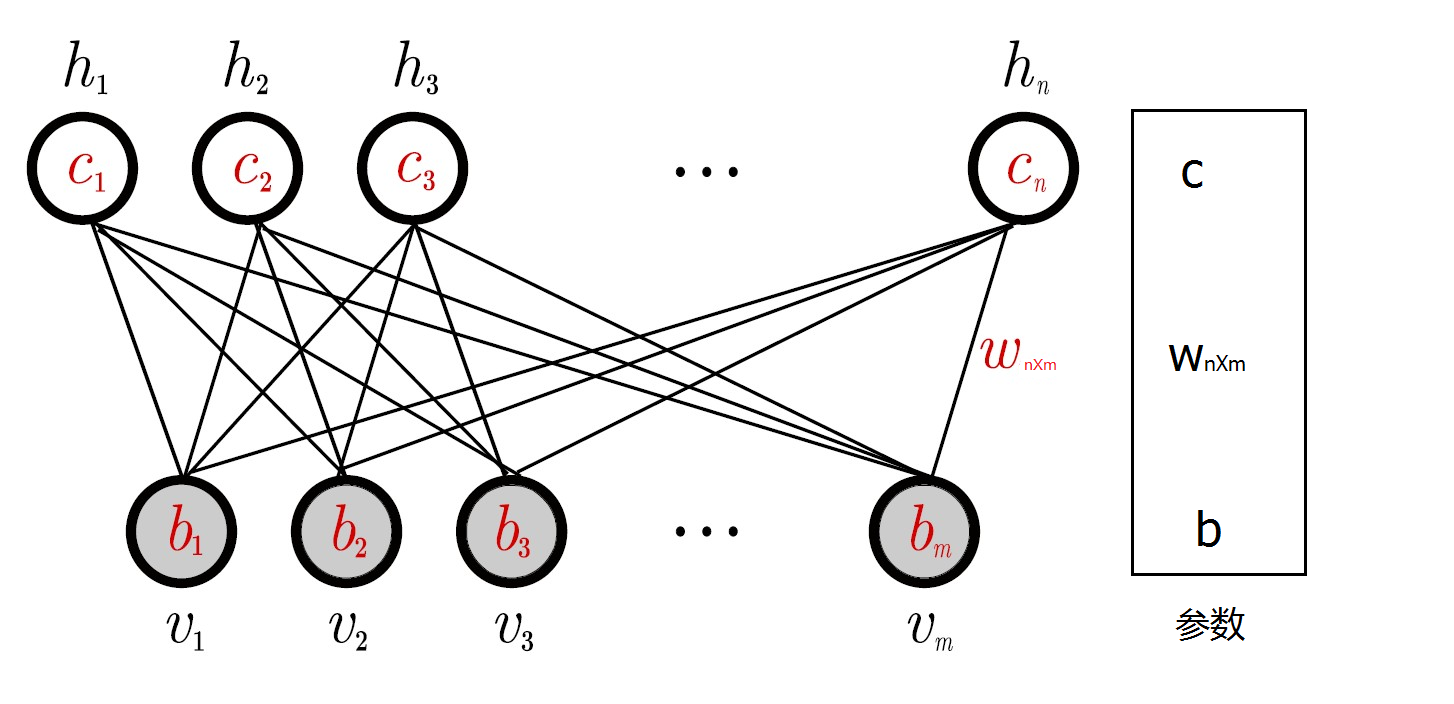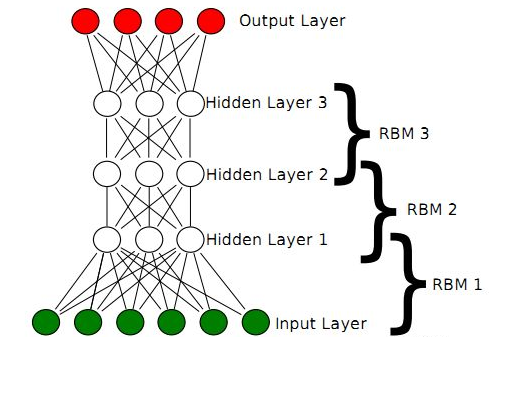# 受限玻尔兹曼机和深度置信网络

### 从玻尔兹曼机到深度置信网络

#### 引言

• 受限玻尔兹曼机（Restricted Boltzmann Machines, RBM）最初是在1986年由Paul Smolensky发明，在Geoffrey Hinton和合作者在2005年左右为其发明了快速学习的算法之后，RBM得以进一步发展。RBM可用于可用于降维、分类、回归、协同过滤、特征学习和主题建模。根据任务，RBM可用于监督学习或无监督学习。

• RBM也可用于深度学习网络。具体而言，深度置信网络（Deep Belief Networks）可以通过“堆叠”RBM并可选地通过梯度下降和反向传播来微调网络的参数。

• 本文将从玻尔兹曼机的结构开始介绍，其后引申至受限玻尔兹曼机，最后介绍由受限玻尔兹曼机堆叠而成的深度置信网络。

#### 1. 玻尔兹曼机E=(i<jwijsisj+iθisi) E = − ( ∑ i < j w i j s i s j + ∑ i θ i s i )

##### 1. 1 玻尔兹曼机节点状态概率

BM的全局能量差值由每个节点的状态差值产生，由下式给出：

$\mathrm{\Delta }{E}_{i}={E}_{i=0}-{E}_{i=1}$

#### 2. 受限玻尔兹曼机

RBM可以表示成一个二分图模型，所有可见层节点和隐藏层节点都有两种状态：处于激活状态时值为1，未被激活状态值为0。这里的0和1状态的意义是代表了模型会选取哪些节点来使用，处于激活状态的节点被使用，未处于激活状态的节点未被使用。节点的激活概率由可见层和隐藏层节点的分布函数计算。如图1所示，一个RBM包含一个由随机的隐含节点构成的隐藏层(一般是伯努利分布)和一个由随机的可见节点构成的可见层(一般是伯努利分布或高斯分布)。

RBM有如下性质：

##### 2.1 受限玻尔兹曼机参数学习

RBM中， v v $v$表示所有可见单元，$h$$h$表示所有隐单元。要想确定该模型，只要能够得到模型三个参数 θ={W,A,B} θ = { W , A , B } $\theta=\{W,A,B\}$即可。分别是权重矩阵 W W $W$，可见层单元偏置$A$$A$，隐藏层单元偏置 B B $B$

$W=\left\{{w}_{i,j}\in {R}^{n×m}\right\}$$W=\{w_{i,j}\in R^{n\times m}\}$ ，其中 Wi,j W i , j $W_{i,j}$表示第 i i $i$个可见单元和第$j$$j$个隐单元之间的权值
A={aiRm} A = { a i ∈ R m } $A=\{a_i\in R^m\}$ ，其中 ai a i $a_i$表示第 i i $i$个可见单元的偏置阈值
$B=\left\{{b}_{j}\in {R}^{n}\right\}$$B=\{b_j\in R^n\}$ ，其中 bj b j $b_j$表示第 j j $j$个可见单元的偏置阈值

E(v,h|θ)=i=1naivij=1mbjhji=1nj=1mviWijhj E ( v , h | θ ) = − ∑ i = 1 n a i v i − ∑ j = 1 m b j h j − ∑ i = 1 n ∑ j = 1 m v i W i j h j

P(v,h|θ)=eE(v,h|θ)Z(θ) P ( v , h | θ ) = e − E ( v , h | θ ) Z ( θ )
Z(θ)=v,heE(v,h|θ) Z ( θ ) = ∑ v , h e − E ( v , h | θ )

P(v|θ)=1Z(θ)heE(v,h|θ) P ( v | θ ) = 1 Z ( θ ) ∑ h e − E ( v , h | θ )

1）在给定可见单元的状态时，各隐藏层单元的激活状态之间是条件独立的。此时，第 j j $j$个隐单元的激活概率为：

$P\left({h}_{j}=1|v\right)=\sigma \left({b}_{j}+\sum _{i}{v}_{i}{W}_{ij}\right)$
2）相应的，当给定隐单元的状态时，可见单元的激活概率同样是条件独立的：

P(vi=1|h)=σ(ai+jWijhj) P ( v i = 1 | h ) = σ ( a i + ∑ j W i j h j )##### 2.2 受限玻尔兹曼机模型参数求解

P(v|θ)=1Z(θ)heE(v,h|θ) P ( v | θ ) = 1 Z ( θ ) ∑ h e − E ( v , h | θ ) $P(v|\theta)=\frac{1}{Z(\theta)}\sum_he^{-E({v,h|\theta})}$可知，能量 E E $E$和概率$P$$P$成反比，通过最大化 P P $P$来最小化$E$$E$。最大化似然函数常用方法是梯度上升法，梯度上升法是指按照以下公式对参数进行修改：

θ=θ+μlnP(v)θ θ = θ + μ ∂ l n P ( v ) ∂ θ

lnP(vs)wi,j=P(hi=1|vs)vsjvP(v)P(hi=1|v)vj ∂ l n P ( v s ) ∂ w i , j = P ( h i = 1 | v s ) v j s − ∑ v P ( v ) P ( h i = 1 | v ) v j

lnP(vs)ai=vsivP(v)vi ∂ l n P ( v s ) ∂ a i = v i s − ∑ v P ( v ) v i

lnP(vs)bi=P(hi=1|vs)vP(v)P(hi=1|v) ∂ l n P ( v s ) ∂ b i = P ( h i = 1 | v s ) − ∑ v P ( v ) P ( h i = 1 | v )

##### 2.3 模型训练算法

k步CD算法（CD-k）具体可描述为：对 vS ∀ v ∈ S $\forall v\in S$，取初始值 v(0):=v v ( 0 ) := v $v^{(0)}:=v$，然后执行k次采样：
for t = 1,2,3,……,k:
利用 P(h|v(t1)) P ( h | v ( t − 1 ) ) $P(h|v^{(t-1)})$ 采样出 h(t1) h ( t − 1 ) $h^{(t-1)}$
利用 P(v|h(t1)) P ( v | h ( t − 1 ) ) $P(v|h^{(t-1)})$ 采样出 v(t) v ( t ) $v^{(t)}$

lnP(v)wi,jP(hi=1|v(0))v(0)jP(hi=1|v(k))v(k)j ∂ l n P ( v ) ∂ w i , j ≈ P ( h i = 1 | v ( 0 ) ) v j ( 0 ) − P ( h i = 1 | v ( k ) ) v j ( k )

lnP(v)aiv(0)iv(k)i ∂ l n P ( v ) ∂ a i ≈ v i ( 0 ) − v i ( k )

lnP(v)biP(hi=1|v(0))P(hi=1|v(k)) ∂ l n P ( v ) ∂ b i ≈ P ( h i = 1 | v ( 0 ) ) − P ( h i = 1 | v ( k ) )

##### 2.3.模型的评估#### 3 深度置信网络（1）最底部RBM以原始输入数据进行训练
（2）将底部RBM抽取的特征作为顶部RBM的输入继续训练
（3）重复这个过程训练以尽可能多的RBM层

Hinton提出，这种预训练过程是一种无监督的逐层预训练的通用技术，也就是说，不是只有RBM可以堆叠成一个深度网络，其它类型的网络也可以使用相同的方法来生成网络。

###### 参考文献

https://www.cnblogs.com/jhding/p/5687696.html https://blog.csdn.net/u010223750/article/details/60882390
https://blog.csdn.net/itplus/article/details/19408143
Hinton G. A practical guide to training restricted Boltzmann machines[J]. Momentum, 2010, 9(1): 926.
Bengio Y, Lamblin P, Popovici D, et al. Greedy layer-wise training of deep networks[J]. Advances in neural information processing systems, 2007, 19: 153.
Hinton G E, Osindero S, Teh Y W. A fast learning algorithm for deep belief nets[J]. Neural computation, 2006, 18(7): 1527-1554.
https://en.wikipedia.org/wiki/Restricted_Boltzmann_machine
https://blog.csdn.net/Rainbow0210/article/details/53010694?locationNum=1&fps=1
https://my.oschina.net/u/876354/blog/1626639

11-26
08-1707-25
01-071万+
12-141万+
06-12928
08-21
03-24988
01-022万+
02-142472